# Test: Single Correct MCQs: The p-Block Elements | JEE Advanced

## 62 Questions MCQ Test Class 12 Chemistry 35 Years JEE Mains &Advance Past yr Paper | Test: Single Correct MCQs: The p-Block Elements | JEE Advanced

Description
Attempt Test: Single Correct MCQs: The p-Block Elements | JEE Advanced | 62 questions in 120 minutes | Mock test for JEE preparation | Free important questions MCQ to study Class 12 Chemistry 35 Years JEE Mains &Advance Past yr Paper for JEE Exam | Download free PDF with solutions
QUESTION: 1

### The reddish brown coloured gas formed when nitric oxide is oxidised by air is

Solution: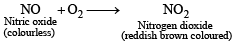QUESTION: 2

### The temporary hardness of water due to calcium carbonate can be removed by adding –

Solution:

Temporary hardness of water is due to presence of bicarbonates of Ca and Mg and it is removed by adding Ca(OH)2 to hard water and precipitating these soluble bicarbonates in the form of insoluble salts.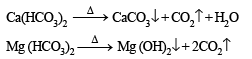QUESTION: 3

### Which of the following is most stable to heat

Solution:

Due to highest bond dissociation energy.

QUESTION: 4

White P reacts with caustic soda. The products are PH3 and NaH2PO2. This reaction is an example of

Solution: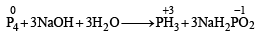It is redox reaction. (i) Oxidation n umber in cr eases dur in g oxidation reaction and decreases during reduction reaction.
(ii) In a neutralisation reaction acids and bases  reacts together to form salt and water.

QUESTION: 5

A solution of KBr is treated with each of the following.

Which one would liberate bromine

Solution:

∵ Cl2 is more reactive than bromine.

QUESTION: 6

Which of the following is coloured

Solution:

All are colourless gases.

QUESTION: 7

Solution:
QUESTION: 8

Ammonia can be dried by

Solution:

None; it reacts with all given compounds. It forms addition compounds with them.
It can be dried over any metal oxide.

QUESTION: 9

HBr and HI reduce sulphuric acid, HCl can reduce KMnO4 and HF can reduce

Solution:

HI and HBr (in that order) are the strongest reducing hydracids and hence they reduce H2SO4. HCl is quite stable and hence is oxidised by strong oxidising agent like KMnO4. HF is not a reducing agent. In the smallest F– ion, the electron which is to be removed during oxidation is closest to the nucleus and therefore most difficult to be removed. Therefore, HF is a poor reducing agent.

QUESTION: 10

Which of the following statements about anhydrous aluminium chloride is correct?

Solution:

AlCl3 exists as a dimer (Al2Cl6). It is a strong Lewis acid as it has an incomplete octet and has a tendency to gain electrons. AlCl3 undergoes hydrolysis easily and forms an acidic solution.
AlCl3 + 3H2O → Al(OH)3 + 3HCl

Option (c) is true that AlCl3 sublimes at 100ºC under vacuum.
AlCl3 is a Lewis acid.

QUESTION: 11

Moderate electrical conductivity is shown by

Solution:

Graphite shows moderate electrical conductivity due to the presence of unpaired or free fourth valence electron on each carbon atom.

QUESTION: 12

Chlorine acts as a bleaching agent only in presence of

Solution:

Bleaching action of chlorine is only in presence of moisture where nascent oxygen is displaced from H2O.
Cl2 + H2O → HCl + HClO (unstable) HClO → HCl + | O |

QUESTION: 13

Nitrogen dioxide cannot be obtained by heating :

Solution:

Only nitrates of heavy metals and lithium decompose on heating to produce NO2.

QUESTION: 14

A gas that cannot be collected over water is :

Solution:

SO2 is highly soluble in water and therefore cannot be collected over water.

QUESTION: 15

The compound which gives off oxygen on moderate heating is :

Solution: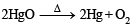QUESTION: 16

The bonds present in N2O5 are :

Solution: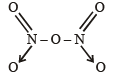The structure clearly shows the presence of covalent and co-ordinate bonds.

QUESTION: 17

Which of the following oxides of nitrogen is a coloured gas?

Solution:

NO2 is reddish brown coloured gas. Rest of the oxides are colourless.

QUESTION: 18

Amongst the trihalides of nitrogen which one is least basic?

Solution:

Least basic trihalogen of nitrogen is NF3 because of the highest electronegativity of fluorine.

QUESTION: 19

Bromine can be liberated from potassium bromide solution by the action of

Solution:

Chlorine is stronger oxidising agent than bromine therefore, chlorine water will liberate bromine from KBr solution.

2 KBr + Cl2 → 2 KCl + Br2

QUESTION: 20

There is no S–S bond in :

Solution:

In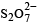there is an S–O–S bond unlike in other.
So S–S bond is absent as can be seen from the structure drawn.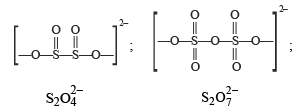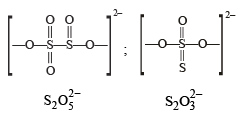QUESTION: 21

In P4O10 each P atom is linked with .......... O atoms

Solution:

In P4O10, each P atom is linked to 4 oxygen atoms as can be confirmed by its structure. It is linked to three oxygen atoms by single bond and with one oxygen atom by double bond. [For structure refer to Q.11 of Section A]

QUESTION: 22

H2SO, cannot be used to prepare HBr from NaBr as it

Solution:

HBr is not prepared by heating NaBr with conc. H2SO4 because HBr is a strong reducing agent and reduce H2SO4 to SO2 and is itself oxidised to bromine.
NaBr + H2SO4 —→ NaHSO4 + HBr H2SO4 + 2HBr —→ SO2 + Br2 + 2H2O

QUESTION: 23

Hydrolysis of one mole of peroxodisulphuric acid produces

Solution: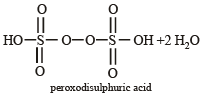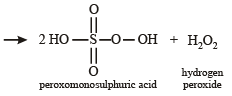QUESTION: 24

Which of the following statements is correct for CsBr3?

Solution:

CsBr3 may be represented as Cs+Br3

QUESTION: 25

KF combines with HF to form KHF2. The compound contains the species.

Solution: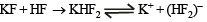QUESTION: 26

Sodium thiosulphate is prepared by

Solution: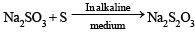QUESTION: 27

Which of the following halides is least stable and has doubtful existence?

Solution:

Due to inert pair effect.

QUESTION: 28

Which one of the following oxides is neutral?

Solution:

CO is an example of neutral oxide.

QUESTION: 29

Which one of the following species is not a pseudohalide?

Solution:

The species called as pseudohalide ions are these are monovalent and made by electronegative atoms. They possess properties similar to halide ion. The corresponding dimers of these pseudohalide ions are called pseudohalogens. RCOO is not is pseudohalide.

QUESTION: 30

One mole of calcium phosphide on reaction with excess water gives

Solution:

Ca3P2 + 6H2O → 3Ca(OH)2 + 2PH3 ; i.e 2 moles of phosphine are produced.

QUESTION: 31

On heating ammonium dichromate, the gas evolved is

Solution:

(NH4)2Cr2O7 → N2 + Cr2O3 + 4H2O

QUESTION: 32

In the commercial electrochemical process for aluminium extraction the electrolyte used is

Solution:

Al2O3 is electrolyte, while  Na3AlF6 is used to decrease the melting point of Al2O3 and to increase the conductivity.

QUESTION: 33

In compounds of type ECl3, where E = B, P, As or Bi, the angles Cl – E– Cl for different E are in the order

Solution:

In BCl3, H = 1/2 (3 + 3 + 0 - 0) = 3 ; sp2 hybridization (bond angle = 120°) similarly PCl3 AsCl3 and BiCl3 are found to have sp3 hybridized central atom with one lone pair of electrons on the central atom. The bond angle < 109°28', since the central atoms belong to the same group, the bond angle of the chlorides decreases as we go down the group. Thus the order of bond angle is,  BCl3 > PCl3 > AsCl3 > BiCl3.

QUESTION: 34

Electrolytic reduction of alumina to aluminium by HallHeroult process is carried out

Solution:

Cryolite reduces the melting temperature.

QUESTION: 35

The number of P – O – P bonds in cyclic metaphosphoric acid is

Solution:

In cyclic metaphosporic acid number of P–O–P bonds is three.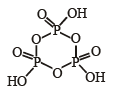QUESTION: 36

Ammonia can be dried by

Solution:

NH3 does not react with CaO while other reacts with NH3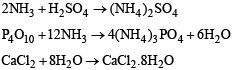QUESTION: 37

The number of S - S bonds in sulphur trioxide trimer (S3O9) is

Solution:

In sulphur trioxide trimer S3O9 (also called g-sulphur trioxide) two sulphur atoms are linked to each other via O atoms, hence there is no S –S bond.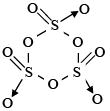QUESTION: 38

Polyphosphates are used as water softening agents because they

Solution: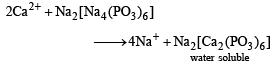QUESTION: 39

For H3PO3 and H3PO4 the correct choice is:

Solution:

The structure of H3PO3 is as follows: There are only two –OH groups and hence dibasic. The oxidation number of P in this acid is +3. Whereas P can have +5 oxidation state also. Therefore, H3PO3 can be oxidised which means H3PO3 is a reducing agent.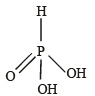QUESTION: 40

H3BO3 is:

Solution:

The central boron atom in boric acid, H3BO3 is electrondeficient.
NOTE : Boric acid is a Lewis acid with one p–orbital vacant. There is no d-orbital of suitable energy in boron atom. So, it can accommodate only one additional electron pair in its outermost shell.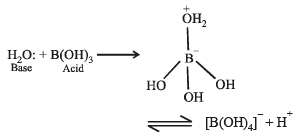QUESTION: 41

(Me)2SiCl2 on hydrolysis will produce

Solution:

TIPS/FORMULAE :
It appears at the first sight that Me2SiCl2 on hydrolysis will produce Me2Si(OH)2 which ultimately upon loss of water, will form Me2Si = O.
But silicon atom, because of its very large size in comparison to oxygen, is unable to form π-bond. Thus, the product of hydrolysis is polymeric in nature.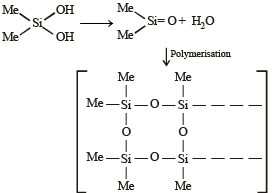QUESTION: 42

Total number of lone pair of electrons in XeOF4 is

Solution:

In XeOF4, Xenon is sp3d2 hybridised and has one lone pair.

QUESTION: 43

The acid having O – O bond is

Solution:

Among oxyacids of sulphur, only Caro’s acid (H2SO5) and Marshall’s acid (H2S2O8) have the O – O linkage.

QUESTION: 44

Pb and Sn are extracted from their chief ores by

Solution:

Tin can be extracted only by carbon reduction method, while lead can be extracted by self as well as carbon reduction method.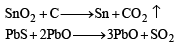QUESTION: 45

Name of the structure of silicates in which three oxygen atoms of [SiO4]4– are shared.

Solution:

Two dimensional sheet structures are formed when three oxygen atoms of each [SiO4]4– tetrahedral are shared.

QUESTION: 46

Which is the most thermodynamically stable allotropic form of phosphorus?

Solution:

The ignition temperature of black phosphorus is highest among all its allotropes, hence is most stable.

QUESTION: 47

Which of the following is not oxidized by O, ?

Solution:

In KMnO4 manganese is already present in its highest possible oxidation state i.e. +7.So no further oxidation is possible.

QUESTION: 48

Blue liquid which is obtained on reacting equimolar amounts of two gases at -30°C is?

Solution: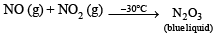QUESTION: 49

When PbO2 reacts with conc. HNO3 the gas evolved is

Solution:

PbO2 is a powerful oxidizing agent and liberate O2 when treated with acids.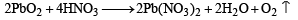QUESTION: 50

How can the following reaction be made to proceed in forward direction?

B(OH)3 + NaOH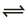NaBO2 + Na[B(OH)4] + H2O

Solution:

cis-1,2-diol for ms ch elated complex ion with th e product, [B(OH)4] causing the reaction to proceed in forward direction.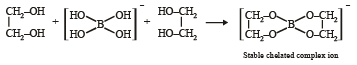QUESTION: 51

The percentage of p-character in the orbitals forming P – P bonds in P4 is

Solution:

In P4, the P–P linkage is formed by sp3–sp3 hybridised orbital overlapping. So the percentage of p-character will be 75%.

QUESTION: 52

Aqueous solution of Na2S2O3 on reaction with Cl2 gives –

Solution:

The following reaction occurs

Na2S2O3 + 4Cl2 + 5H2O → 2 NaHSO4 + 8HCl.

QUESTION: 53

The reaction of P4 with X leads selectively to P4O6. The X is

Solution: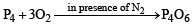Here N2 acts as a diluent and thus retards further oxidation. Reaction of P4 under other three conditions.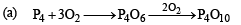(c) In moist air, P4O6 is hydrolysed to form H3PO3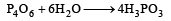(d) In presence of NaOH,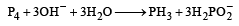QUESTION: 54

Extra pure N2 can be obtained by heating

Solution:

Ba (N3)2 → Ba+ 3N2

QUESTION: 55

Which ordering of compounds is according to the decreasing order of the oxidation state of nitrogen?

Solution: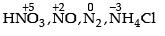QUESTION: 56

The reaction of white phosphorus with aqueous NaOH gives phosphine along with another phosphorus containing compound. The reaction type; the oxidation states of phosphorus in phosphine and the other product are respectively

Solution: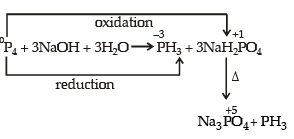QUESTION: 57

The shape of XeO2F2 molecule is

Solution:

XeO2F2 has tr igonal bipyramidal geometry, due to presence of lone pair of electrons on equitorial position, its shape is see-saw.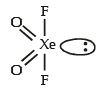QUESTION: 58

Concentrated nitric acid, upon long standing, turns yellow brown due to the formation of

Solution:

The slow decomposition of HNO3 is represented by the eqn.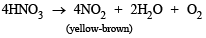QUESTION: 59

The product formed in the reaction of SOCl2 with white phosphorous is

Solution: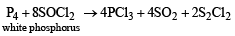QUESTION: 60

Under ambient conditions, the total number of gases released as products in the final step of the reaction scheme shown below is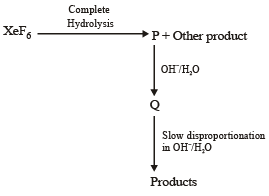Solution: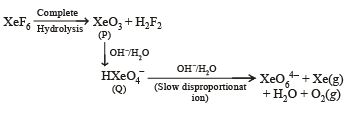QUESTION: 61

The increasing order of atomic radii of the following Group 13 elements is

Solution:

Atomic r adii increases on moving down a gr oup.
However due to poor shielding effect of d orbit, atomic radius of Ga is smaller than Al (anomaly). Thus the correct order is  Ga < Al < In < Tl

QUESTION: 62

In the following reaction sequence in aqueous solution, the species X, Y and Z, r espectively, are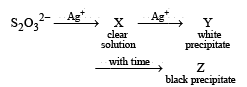Solution: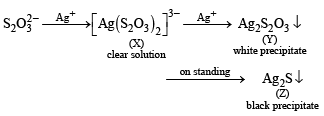So, X, Y and Z are [Ag (S2O3)2]3–, Ag2S2O3 and Ag2S respectively.Use Code STAYHOME200 and get INR 200 additional OFF Use Coupon Code